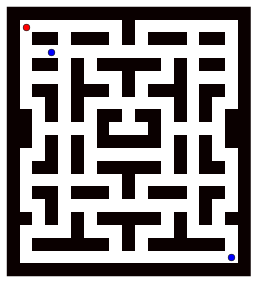Warning

## T4a-greedy - Greedy policy in pursuit-evasion

The main task is to implement a simple greedy policy for robotics pursuit-evasion game.

 Deadline 13. December 2020, 23:59 PST Points 3 Label in BRUTE t4a-greedy Files to submit archive with Player.py Minimal content of the archive: Player.py Resources T4-resource-pack

#### Assignment

In file player/Player.py in function greedy_policy implement the $\epsilon$-greedy policy decision making for pursuit-evasion game. The pursuit-evasion problem is a problem in computer science where a set of pursuers is trying to catch a set of evaders.In greedy policy, the next-best state is selected in each discrete step of the game simulation without considering longer prediction horizon.

• For evaders select the most distant node to the closest pursuer (closest after the move, not before)
• For pursuers select the closest node to the closest evader

In $\epsilon$-greedy policy, the $\epsilon$ parameter gives the probability, with which the robot selects the next-step according to the above strategy. I.e., when $\epsilon=1$ the robot selects the next step according to the rules described above, when $\epsilon=0$ the robot selects its next step randomly.

In each step of the game, the player has to move all its robots for a single step (4-neighborhood).

The greedy_policy function has the following prescription:

    def greedy_policy(self, gridmap, evaders, pursuers, epsilon=1):
"""
Method to calculate the greedy policy action

Parameters
----------
gridmap: GridMap
Map of the environment
list of coordinates of evaders in the game (except the player's robots, if he is evader)
pursuers: list((int,int))
list of coordinates of pursuers in the game (except the player's robots, if he is pursuer)
epsilon: float (optional)
optional epsilon-greedy parameter
"""

The purpose of the function is to internally update the self.next_robots variable, which is a list of (int, int) robot coordinates based on the current state of the game, given gridmap grid map of the environment and the player's role self.role. The player is given the list evaders of all evading robots in the game other than his robots and the list of pursuers of all pursuing robots in the game other than his robots. I.e., the complete set of robots in game is given as the union of evaders, pursuers and self.robots.

During the gameplay, each player is asked to update their intention for the next move coded in the self.next_robots variable by calling the calculate_step function. Afterward, the step is performed by calling the take_step function followed by the game checking each step, whether it complies to the rules of the game.

The game ends after a predefined number of steps or when all the evaders are captured.

#### Approach

The recommended approach to the $\epsilon$-greedy policy implementation is following:

• Given the epsilon paramater return either random move or
• For each robot, evaluate its options of movement and select the best one (given the $\epsilon$ parameter)
• If the player's role is pursuer, calculate the distance from each option to each evader
• If the player's role is evader, calculate the distance from each option to each pursuer
• The distance is given as the number of steps between the position of evader/pursuer and the optional position of the robot. For that you may use the precalculated distance matrix accessible using the dist(start, goal) function of the GridMap class. (The values have been calculated using the Floyd-Warshall algorithm)
• Based on the player's role, select the best option. Note, the evaders select the most distant node to the closest pursuer and pursuers select the closest node to the closest evader.

#### Evaluation

Automatic evaluation is prepared for this task. It checks only the greedy part of the policy so make sure to upload the player with $\epsilon$=1. If the wrong move is encountered in the game, upload system will show you the wrong move, with the state of the game, where it was encountered.

#### Example behavior of two greedy players